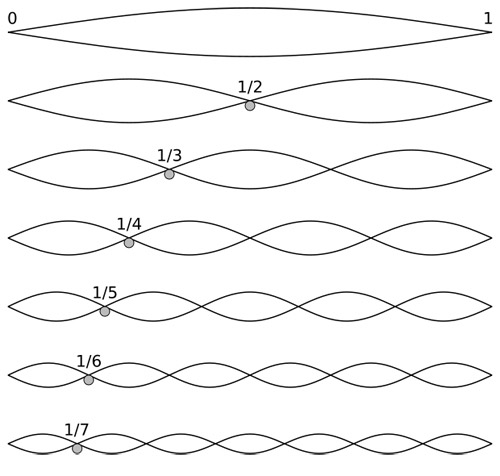A# fundamentalFigure 1. Vibration and standing waves in a string, The fundamental and first 6 overtones.Figure 2. Harmonic series based on G.

The fundamental, also called the fundamental frequency, or first harmonic (see harmonics), is the lowest component in a harmonic series, or the basic frequency of a given note. The fundamental is the loudest element in a note and the one by which the pitch of the note is identified.

In the case of a guitar string, for example, the fundamental is the sound generated by the string vibrating in a single loop along its entire length (see Figure 1).

Taking the note G as the fundamental note, the harmonic series, carried toa reasonable height, would be as shown in Figure 2.